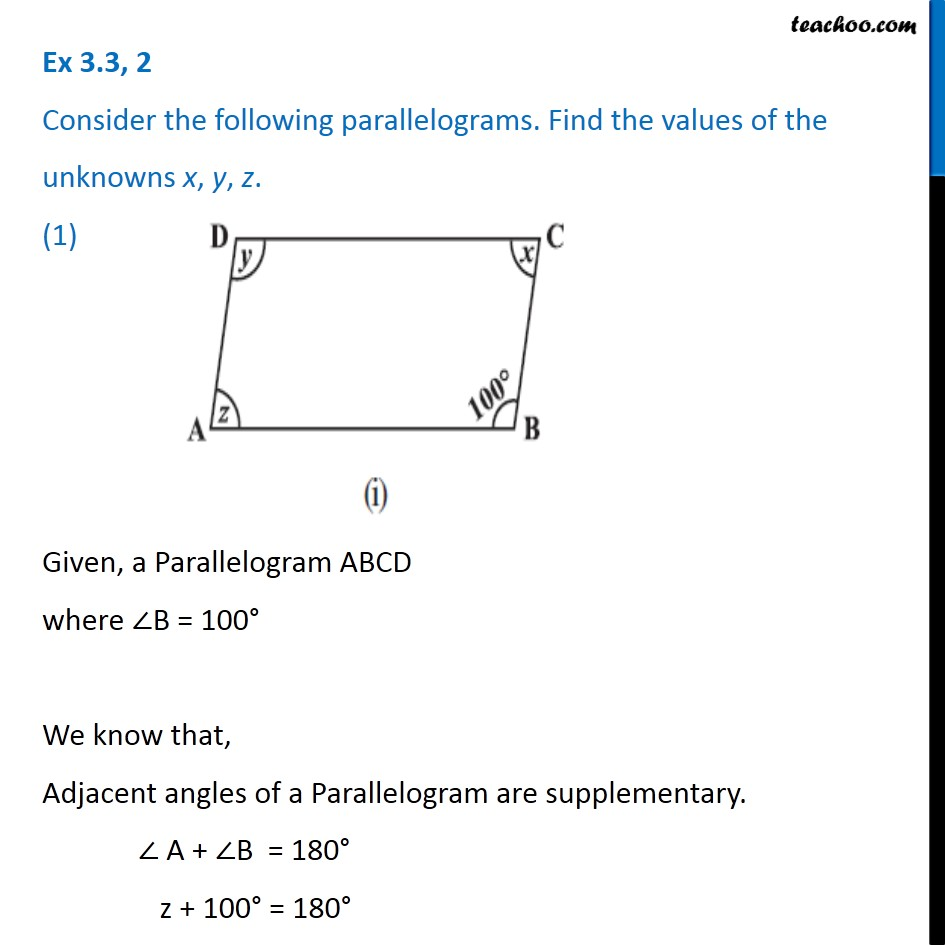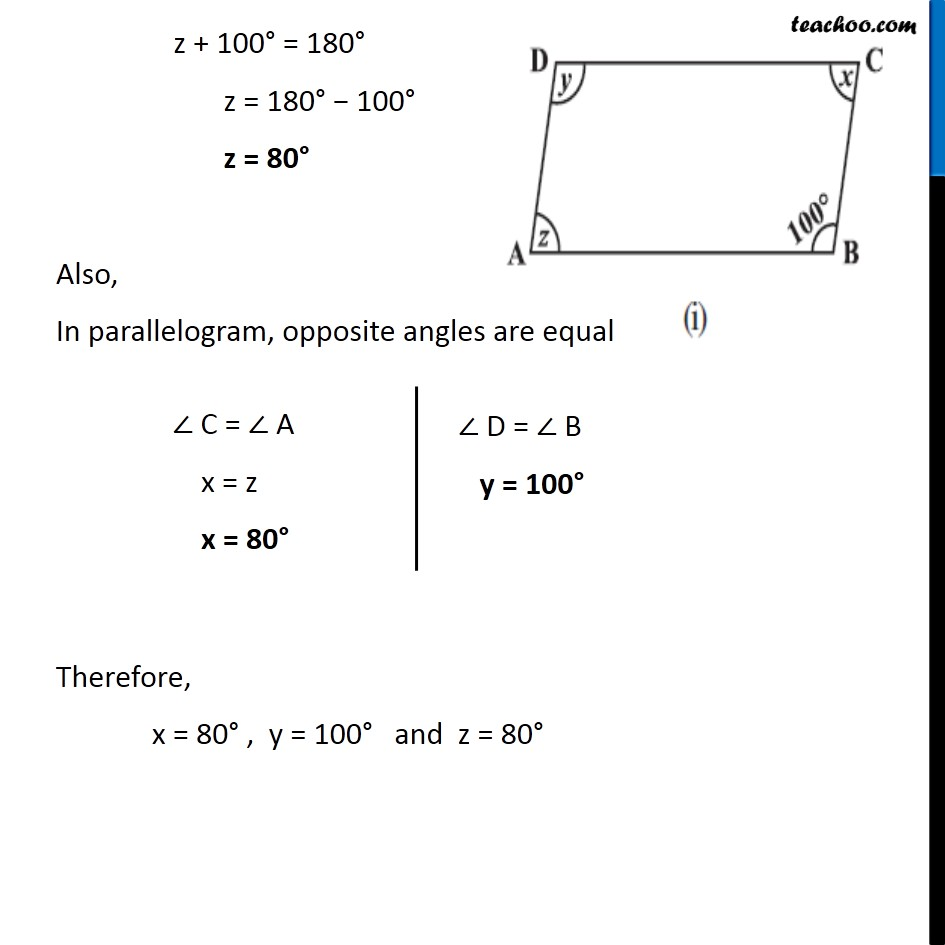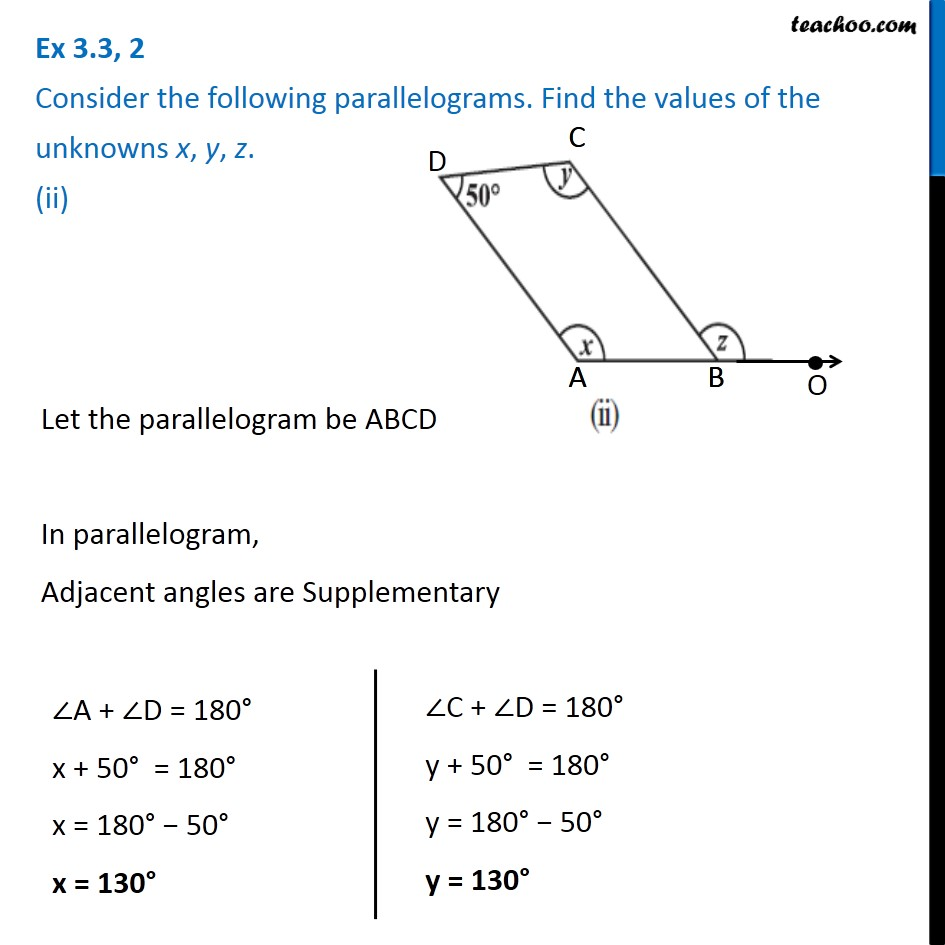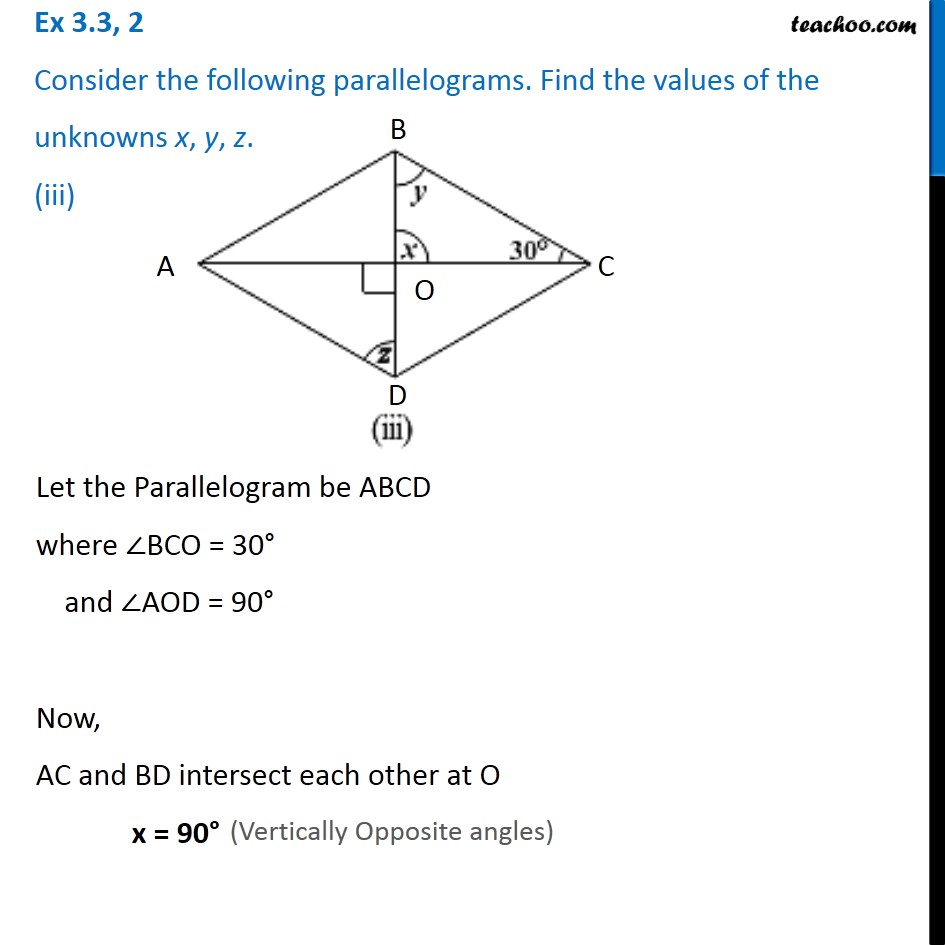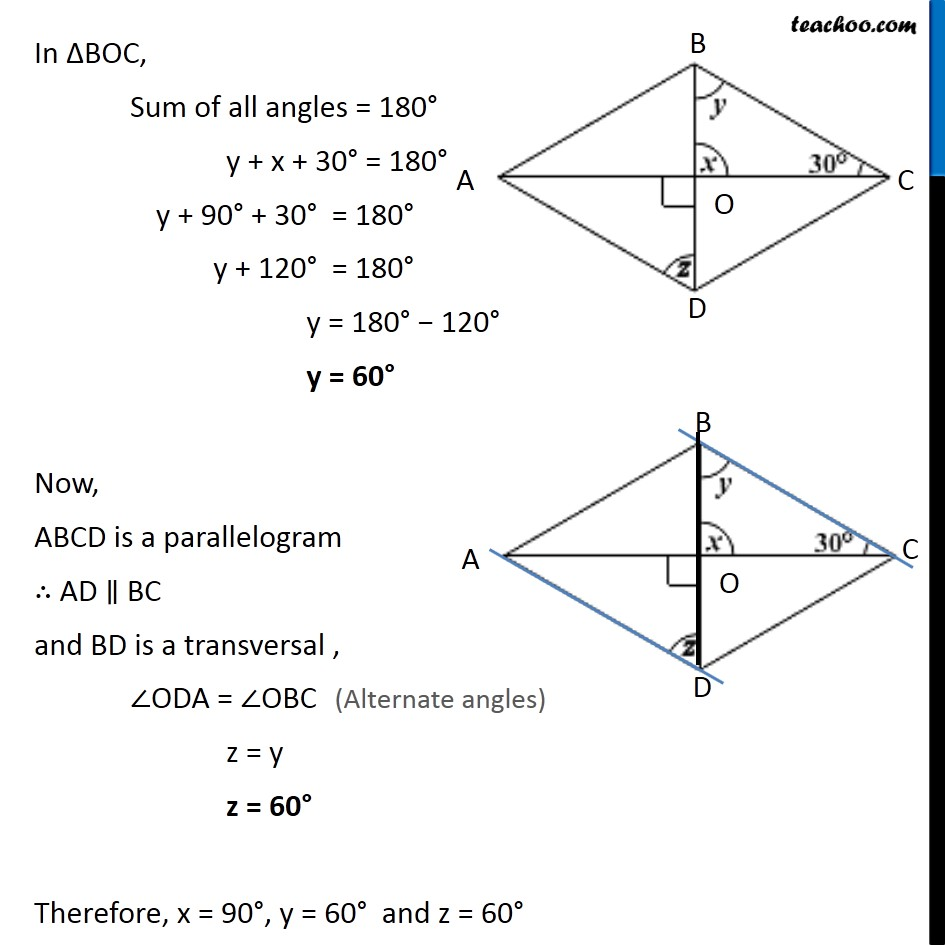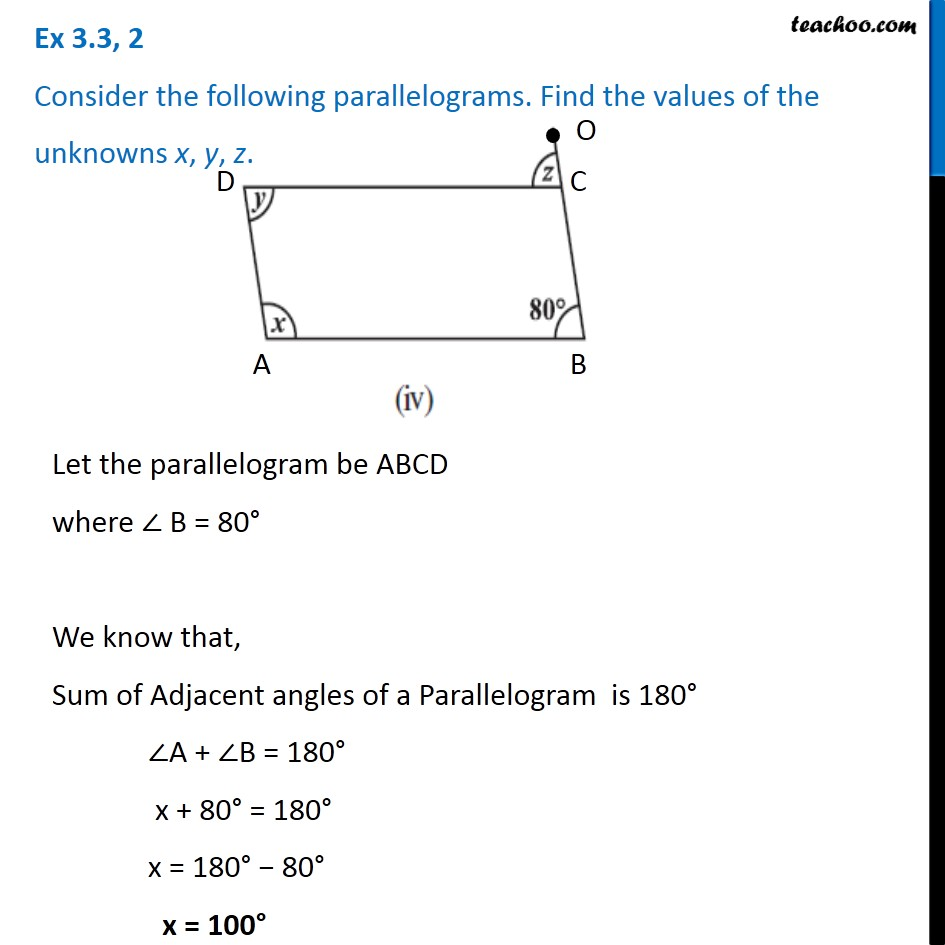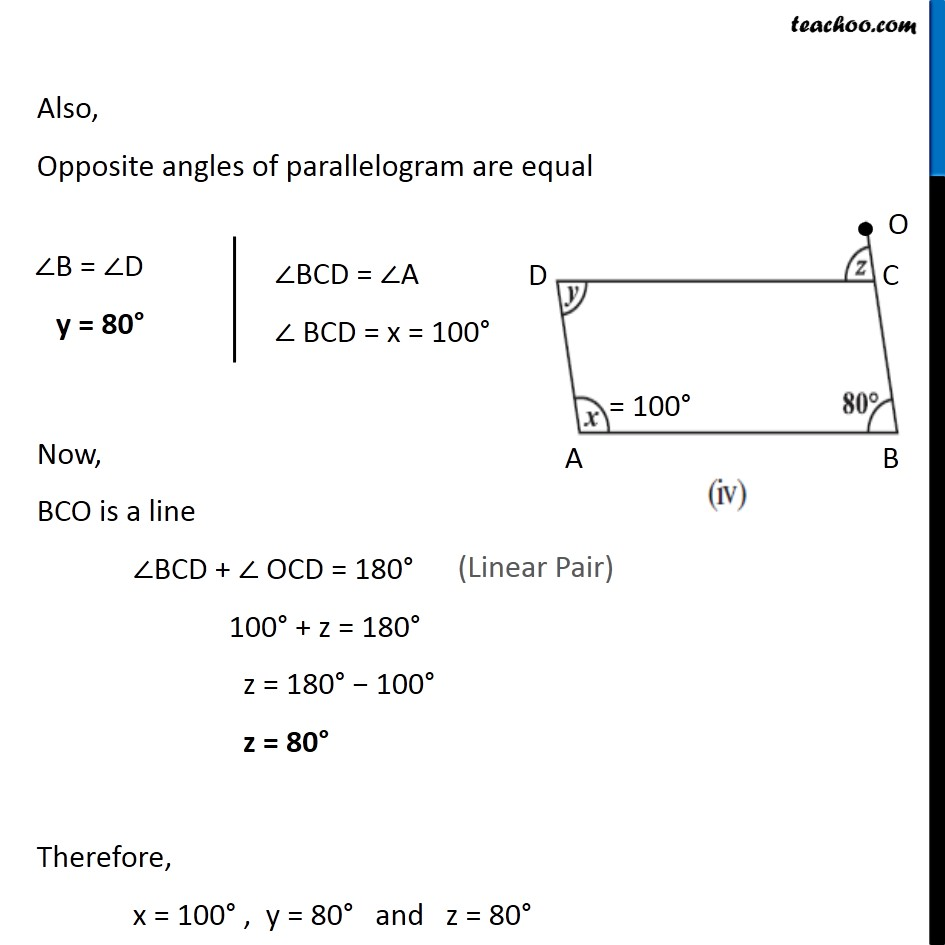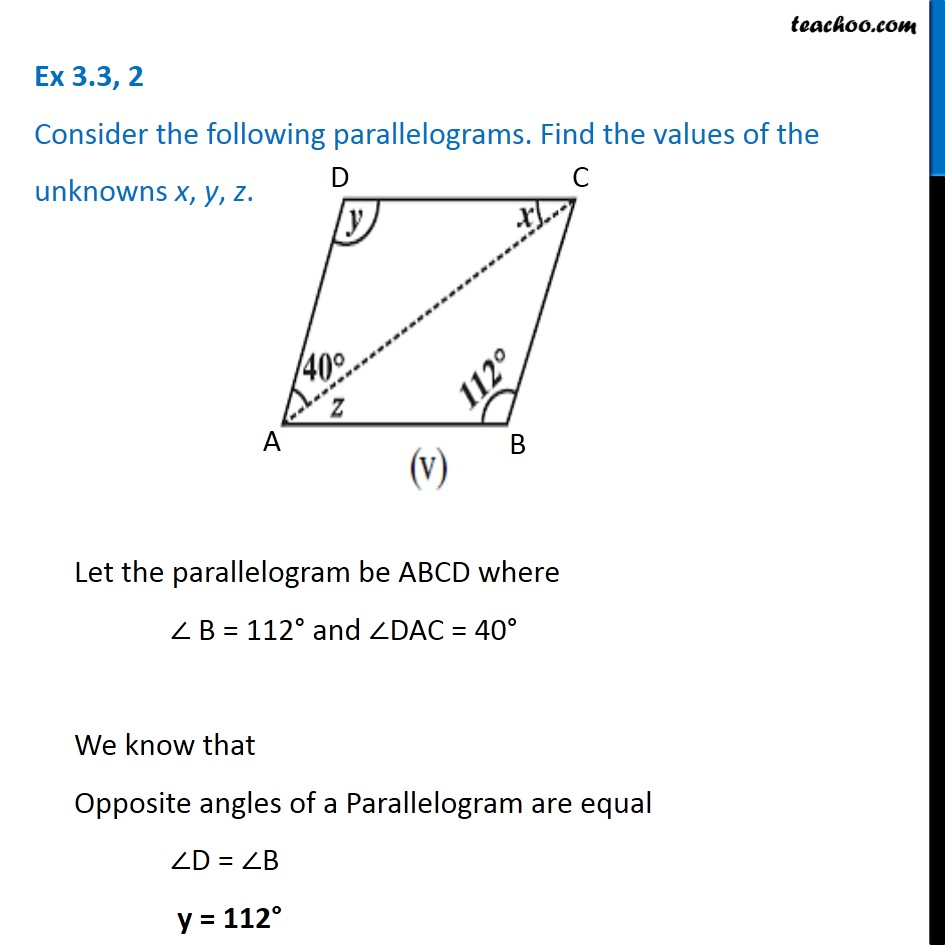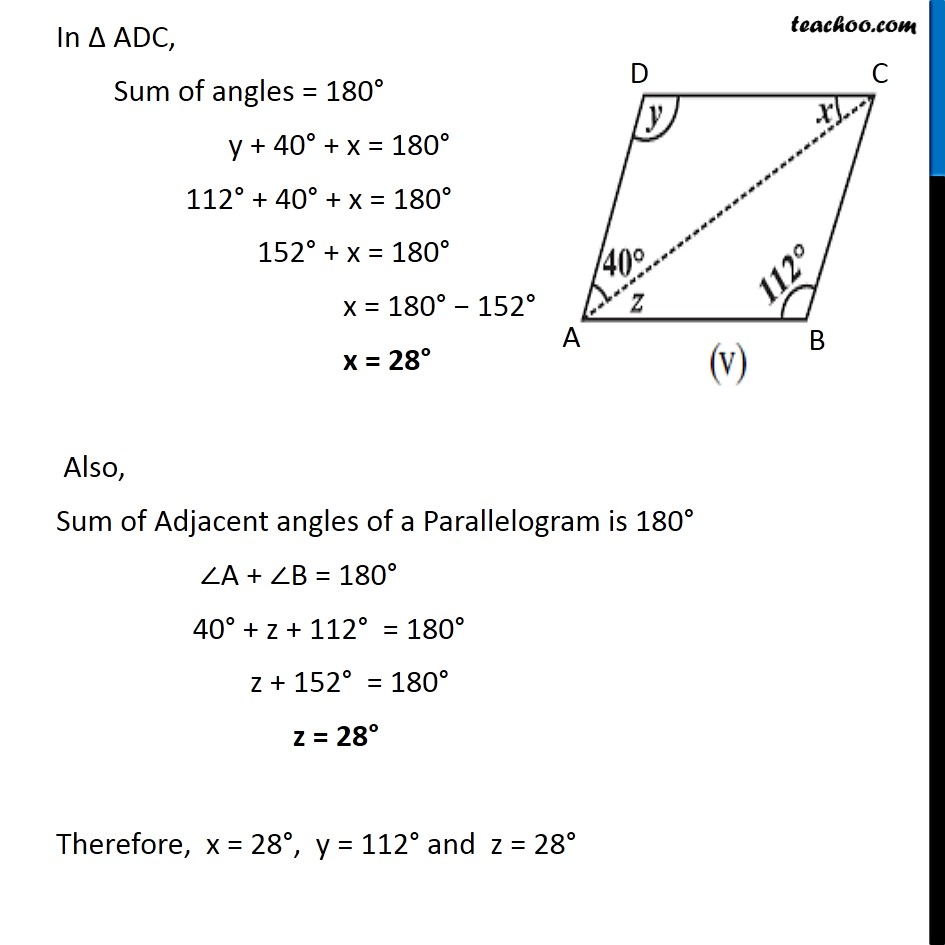Subscribe to our Youtube Channel - https://you.tube/teachoo

1. Chapter 3 Class 8 Understanding Quadrilaterals
2. Serial order wise
3. Ex 3.3

Transcript

Ex 3.3, 2 Consider the following parallelograms. Find the values of the unknowns x, y, z. (1) Given, a Parallelogram ABCD where ∠B = 100° We know that, Adjacent angles of a Parallelogram are supplementary. ∠ A + ∠B = 180° z + 100° = 180° z + 100° = 180° z = 180° − 100° z = 80° Also, In parallelogram, opposite angles are equal Therefore, x = 80° , y = 100° and z = 80° Ex 3.3, 2 Consider the following parallelograms. Find the values of the unknowns x, y, z. (ii) Let the parallelogram be ABCD In parallelogram, Adjacent angles are Supplementary ∠A + ∠D = 180° x + 50° = 180° x = 180° − 50° x = 130° ∠C + ∠D = 180° y + 50° = 180° y = 180° − 50° y = 130° Also, Opposite angles of a Parallelogram are equal ∠ABC = ∠ D ∠ ABC = 50° Now, ABO is a line So, by linear pair ∠ ABC + z = 180° 50° + z = 180° z = 180° − 50° z = 130° Therefore, x = 130° , y = 130° and z = 130° Ex 3.3, 2 Consider the following parallelograms. Find the values of the unknowns x, y, z. (iii)Let the Parallelogram be ABCD where ∠BCO = 30° and ∠AOD = 90° Now, AC and BD intersect each other at O x = 90° In ∆BOC, Sum of all angles = 180° y + x + 30° = 180° y + 90° + 30° = 180° y + 120° = 180° y = 180° − 120° y = 60° Now, ABCD is a parallelogram ∴ AD ∥ BC and BD is a transversal , ∠ODA = ∠OBC z = y z = 60° Therefore, x = 90°, y = 60° and z = 60° Ex 3.3, 2 Consider the following parallelograms. Find the values of the unknowns x, y, z. Let the parallelogram be ABCD where ∠ B = 80° We know that, Sum of Adjacent angles of a Parallelogram is 180° ∠A + ∠B = 180° x + 80° = 180° x = 180° − 80° x = 100° Also, Opposite angles of parallelogram are equal ∠B = ∠D y = 80° ∠BCD = ∠A ∠ BCD = x = 100°Now, BCO is a line ∠BCD + ∠ OCD = 180° 100° + z = 180° z = 180° − 100° z = 80° Therefore, x = 100° , y = 80° and z = 80°

Ex 3.3